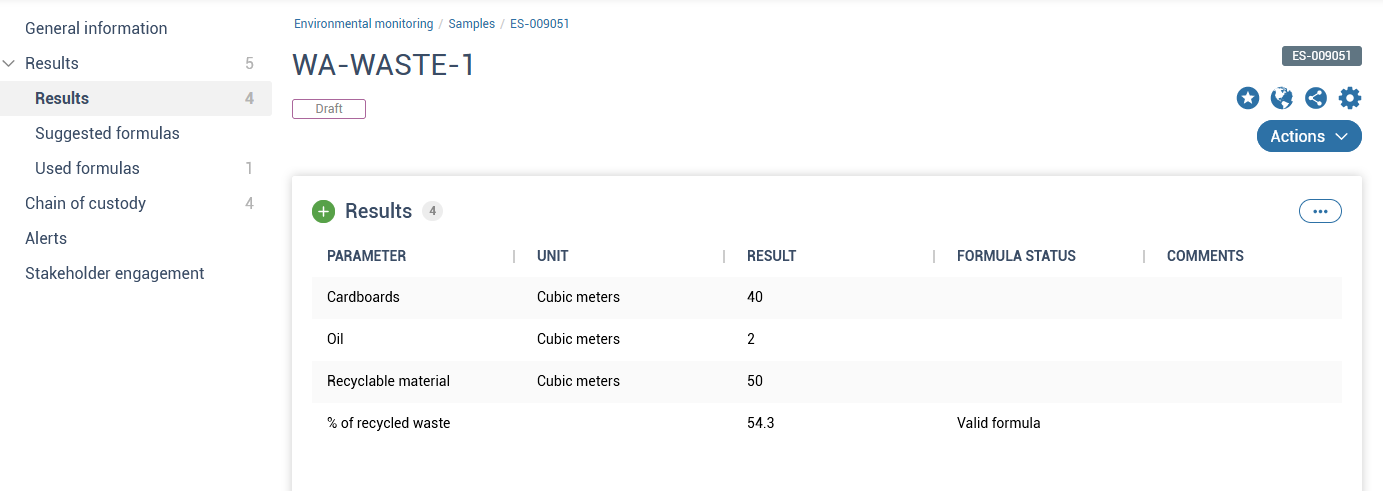# How to work with complex parameters

The calculated parameters will appear in the Results section of a sample. If the "Add to results automatically" box has been ckecked when creating the calculated parameter, this parameter is automatically added to the Results section. If not, it has to manually be added (see Suggested formulas section).Results of calculated values:

• can be removed but not modified
• are updated if results of their parameters or the formula change
• Formula status is marked as
- Incomplete formula if one or more parameters results are missing
- Invalid formula if there is an error (e.g Division by a parameter with zero value)
- Valid formula if the formula and its parameters are valid